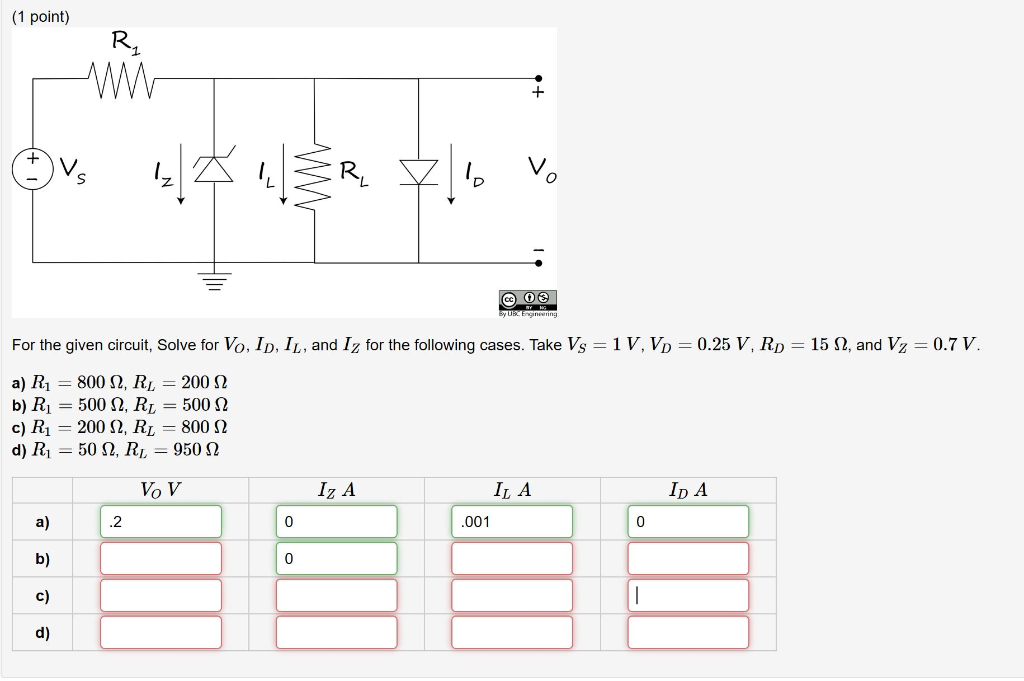# Question For the given circuit, Solve for VO , ID , IL , and IZ for the following cases. Take VS=1 V , VD=0.25 V , RD=15Ω , and VZ=0.7 V .a) R1=800 Ω , RL=200 Ω b) R1=500Ω , RL=500 Ω c) R1=200Ω , RL=800Ω d) R1=50Ω , RL=950Ω (1 point) Ov: 141 ŠR lo vo @ 0 For the given circuit, Solve for Vo, ID, IL, and Iz for the following cases. Take Vs = 1V, Vp = 0.25 V, Rp = 15 12, and V2 = 0.7 V. a) Ri = 800 12, R = 200 12 b) R1 = 500 12, RL = 500 12 c) R1 = 200 S2, RL = 800 32 d) R1 = 502, RL = 950 22 VOV Iz A ILA Ip A .001 /n(1 point) = For the given circuit, Solve for Vo, Ip, Iy,, and Iz, for the following cases. Take Vg = 1 V, Vp = 0.25 V, Rp = 15 Q, and Vz =0.7V. a) R; = 8000, Ry = 2002 b) R, = 500, Ry = 5000 c) R; = 2002, Ry = 8002 d) Ry = 500, Ry, = 9500 VoV IZA ILA IpA a) b) | | °) | | q)WYYCBR The Asker · Electrical Engineering

# For the given circuit, Solve for VO , ID , IL , and IZ for the...

For the given circuit, Solve for VO , ID , IL , and IZ for thefollowing cases. Take VS=1 V , VD=0.25 V , RD=15Ω , and VZ=0.7 V.a) R1=800 Ω , RL=200 Ω
b) R1=500Ω , RL=500Ω
c) R1=200Ω ,RL=800Ω
d) R1=50Ω ,RL=950Ω

(1 point) Ov: 141 ŠR lo vo @ 0 For the given circuit, Solve for Vo, ID, IL, and Iz for the following cases. Take Vs = 1V, Vp = 0.25 V, Rp = 15 12, and V2 = 0.7 V. a) Ri = 800 12, R = 200 12 b) R1 = 500 12, RL = 500 12 c) R1 = 200 S2, RL = 800 32 d) R1 = 502, RL = 950 22 VOV Iz A ILA Ip A .001
Transcribed Image Text: (1 point) = For the given circuit, Solve for Vo, Ip, Iy,, and Iz, for the following cases. Take Vg = 1 V, Vp = 0.25 V, Rp = 15 Q, and Vz =0.7V. a) R; = 8000, Ry = 2002 b) R, = 500, Ry = 5000 c) R; = 2002, Ry = 8002 d) Ry = 500, Ry, = 9500 VoV IZA ILA IpA a) b) | | °) | | q)
More
Transcribed Image Text: (1 point) = For the given circuit, Solve for Vo, Ip, Iy,, and Iz, for the following cases. Take Vg = 1 V, Vp = 0.25 V, Rp = 15 Q, and Vz =0.7V. a) R; = 8000, Ry = 2002 b) R, = 500, Ry = 5000 c) R; = 2002, Ry = 8002 d) Ry = 500, Ry, = 9500 VoV IZA ILA IpA a) b) | | °) | | q)